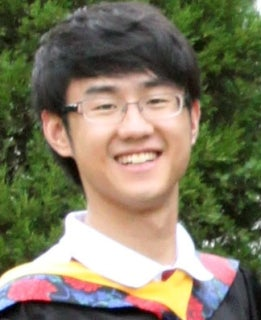# Wei (David) DaiResume
Wei_Dai_CV1.61 KB
Education
 Ph.D., Rensselaer Polytechnic Institute, (2014) B.S., Zhejiang University, (2010)
Research Interests

As a PhD student in Dr. Juergen Hahn's group, my research interests include mathematical modeling, model predictive control, and application of large-scale optimization algorithm to complex dynamic systems.

1. The mathematical modeling work includes: i) the development of dynamic model described by ordinary differential equations (ODEs) or differential algebraic equations (DAEs) based on the first principles, e.g., component balance, energy balance, and the chemical kinetics; ii) the identification of transfer function models from the input-output response; iii) parameter sensitivity analysis of the dynamic model under uncertainty via hierarchical clustering and dynamic optimization.
2. The MPC research that I have conducted involves developing detailed dynamic models, controller development and implementation. This area includes application of the single-MPC-controller strategies to linear dynamic systems and multiple-MPC-controller strategies on nonlinear dynamic system after piecewise linearization. One aspect of this work is implementation of the MPC strategies on embedded hardware.
3. The application of large-scale optimization algorithm includes: i) discretization of ODEs via different collocation methods thus transforming the dynamic optimization problem into a large-scale nonlinear programing problem (NLP); ii) parameter estimation of large-size nonlinear dynamic system; iii) determination of the optimal input profiles of dynamic system for process control and optimal experiment design.
Current Employer

Rockwell Automation